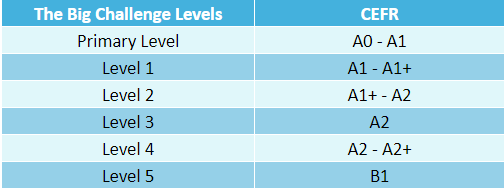Benelux

# Assessment

The assessment

The quiz questions are divided into 3 categories: easy questions, intermediate questions and difficult questions.
The number of questions in each category, as well as the number of points awarded for a question in each category.

All corrections are made by The Big Challenge.
For each of the 6 levels, three rankings will be established: national, departmental or regional and by school.

Contest
Number of questions   55 questions
• 25 easy questions (value of 4 points)
• 15 intermediate question (value of 5 points)
• 15 difficult questions (value of 6 points)
• If a category 1 question is answered incorrectly, the 4 points are not awarded and 1 point is lost.
• If a category 2 question is answered incorrectly, 5 points are not awarded and 1.25 points are lost.
• If a category 3 question is answered incorrectly, the 6 points are not awarded and 1.50 points are lost.
Points in absence of response   If no answer is given, the question is scored 0 points. If more than one answer is given, the question is also scored 0 points.
Bonus points   To avoid the risk of obtaining a negative grade or a grade equal to 0, the grade obtained by adding all the points is increased by 85 points. Thus, each student’s grade will be between 18.75 and 350 points.
Example
 The answer to a 4-point question is correct. + 4 The answer to a 4-point question is incorrect. – 1 The answer to a 5-point question is correct. + 5 The answer to a 5-point question is incorrect. – 1,25 No answer to a question 0 The answer to a 6-point question is correct. + 6 The answer to a 6-point question is incorrect. – 1,50 Two answers are given to the same question 0 Total 11,25 points

11,25 + 85 = 96,25 points

For this example, the final score would be 96.25 out of 350.

How difficult are the questionnaires?

Between primary and secondary there are 6 levels of questionnaires corresponding to each school level. The questionnaires are based on the CEFR.

You will find our recommendations below:If you have any questions regarding the levels, please do not hesitate to contact us and we will be happy to inform you.

What should students do if they don't know the answer to a question?

If you are able to eliminate one or two "wrong answers" and then hesitate between the remaining two, you should answer at random. Otherwise, it's better not to answer.

If you apply this method throughout the questionnaire, you're bound to win in the end. A student who fills in an entire grid at random will have a final mark close to zero with our marking system. With a one in four chance of getting it right (and therefore scoring 4 points) and losing a quarter of that score (1 point) every time they get an answer wrong, they will statistically arrive at a total close to zero.

On the other hand, a student who is not sure which of the four possible answers is right, but who is able to eliminate two wrong answers by thinking, for example, will be favoured because he will have a 50/50 chance of getting it right and will still only lose one point if he is wrong. But it's only because they've thought through the different possibilities first that they'll have a better chance.

And ultimately that's what we want: for students to learn to think before they answer!